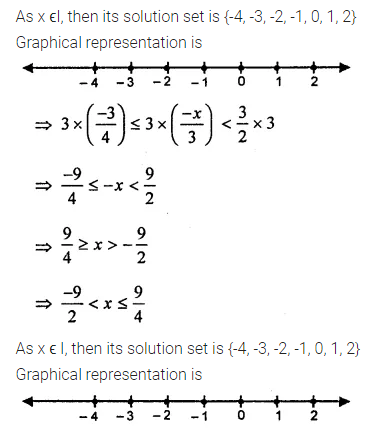# ML Aggarwal Class 8 Solutions for ICSE Maths Chapter 12 Linear Equations and Inequalities in one Variable Check Your Progress

## ML Aggarwal Class 8 Solutions for ICSE Maths Chapter 12 Linear Equations and Inequalities in One Variable Check Your Progress

Question 1.
Solve the following equations:Solution: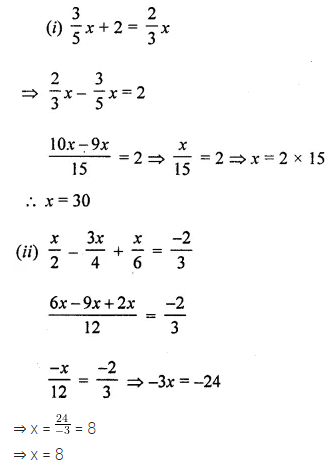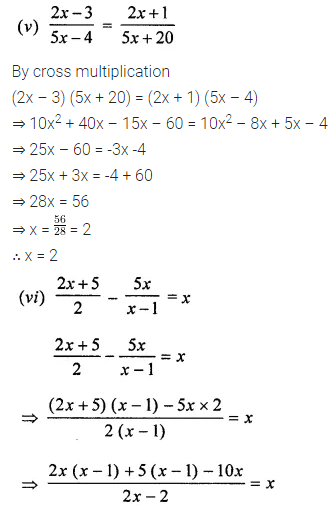Question 2.
The sum of three consecutive multiples of 11 is 363. Find these multiples.
Solution: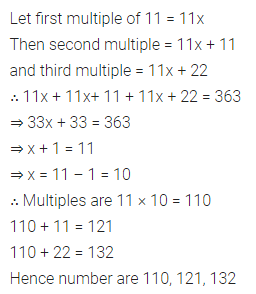Question 3.
Sum of two numbers is 95. If one exceeds the other by 15, find the numbers.
Solution: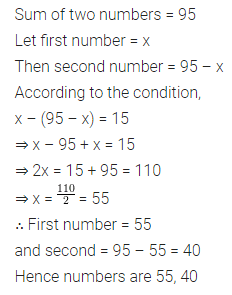Question 4.
One-half of a number is equal to one-third of its succeeding number. Find the first number.
Solution:Question 5.
The numerator of a rational number is 8 less than its denominator. If the numerator is increased by 2 and denominator is decreased by 1, the number obtained is $$\frac{1}{2}$$. Find the number.
Solution:Question 6.
The present ages of Rohit and Mayank are in the ratio 11 : 8. 8 years later the sum of their ages will be 54 years. What are their present ages?
Solution: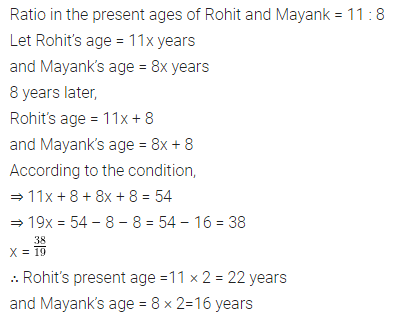Question 7.
A father’s age is 3 times the sum of ages of his two sons. Five years later he will be twice the sum of ages of his two sons. Find the present age of the father.
Solution: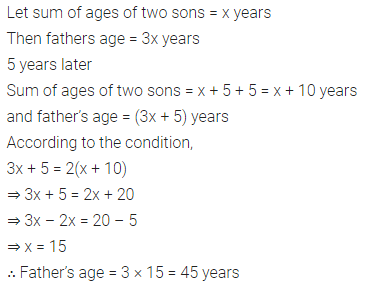Question 8.
The digits of a two-digit number differ by 7. If the digits are interchanged and the resulting number is added to the original number we get 121. Find the original number.
Solution:Question 9.
The ten’s digit of a two-digit number exceeds it’s unit’s digit by 5. When digits are reversed, the new number added to the original number becomes 99. Find the original number.
Solution:Question 10.
Sonia went to a bank with ₹2,00,000. She asked the cashier to give her ₹500 and ₹2000 currency notes in return. She got 250 currency notes in all. Find the number of each kind of currency notes.
Solution: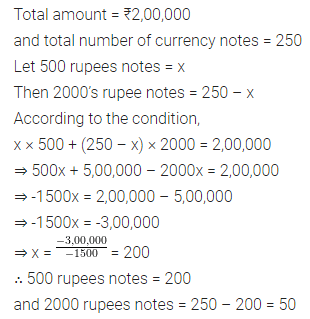Question 11.
Ajay covers a distance of 240 km in $$4 \frac{1}{4}$$ hours. Some part of the journey was covered at the speed of 45 km/h and the remaining at 60 km/h. Find the distance covered by him at the rate of 60 km/h.
Solution: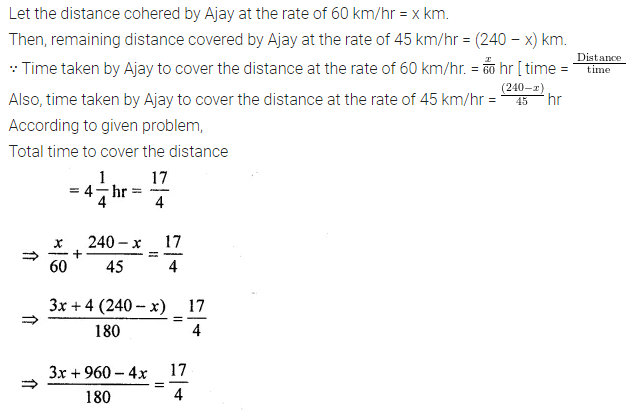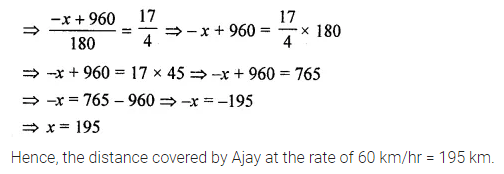Question 12.
If x ϵ {even integers), represent the solution set of the inequation -5 ≤ x < 5 on a number line.
Solution:Question 13.
Solve the following inequality and graph its solution on a number line:Solution: